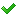•闪存
•博客
• 发言小组
• 投递新闻
• 提问博问
• 添加收藏
• 发布招聘
•文库

3

1

1

##50js function中如何获取list的值

function sum(){ var a = \$('').val(); var b = \$('').val(); var sum= a * b; \$('#total').val(sum); } <t